# Statue

On the pedestal high 4 m is statue 2.7 m high. At what distance from the statue must observer stand to see it in maximum viewing angle? Distance from the eye of the observer from the ground is 1.7 m.

Correct result:

x =  3.39 m

#### Solution: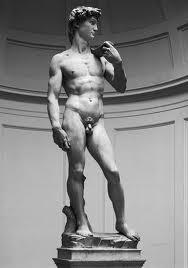We would be pleased if you find an error in the word problem, spelling mistakes, or inaccuracies and send it to us. Thank you!Tips to related online calculators
Looking for a statistical calculator?
Most natural application of trigonometry and trigonometric functions is a calculation of the triangles. Common and less common calculations of different types of triangles offers our triangle calculator. Word trigonometry comes from Greek and literally means triangle calculation.

## Next similar math problems:

• SSA and geometryThe distance between the points P and Q was 356 m measured in the terrain. The PQ line can be seen from the viewer at a viewing angle of 107° 22 '. The observer's distance from P is 271 m. Determine the viewing angle of P and observer.Average climb of the road is given by ratio 1:15. By what angle road average climb?
• Map - climb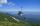On the map of High Tatras in scale 1:11000 are cable car stations in the Tatranska Lomnica and in the Skalnate Pleso with distance 354.6 mm. Altitude of this stations are 949 m and 1760 m. What is average angle of climb of this cable car track?
• Tangents to ellipseFind the magnitude of the angle at which the ellipse x2 + 5 y2 = 5 is visible from the point P[5, 1] .
• BevelI have bevel in the ratio 1:6. What is the angle and how do I calculate it?
• Viewing angleThe observer sees a straight fence 60 m long at a viewing angle of 30°. It is 102 m away from one end of the enclosure. How far is the observer from the other end of the enclosure?
• Traffic signThere is a traffic sign for climbing on the road with an angle of 7%. Calculate at what angle the road rises (falls).
• TreeHow tall is the tree that observed in the visual angle of 52°? If I stand 5 m from the tree and eyes are two meters above the ground.
• Hot air balloon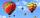The center of the balloon is at an altitude of 600 m above the ground (AGL). From habitat on earth is the center of the balloon to see in elevation angle 38°20' and the balloon is seen from the perspective of angle 1°16'. Calculate the diameter of the bal
• Shopping malls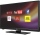The chain of department stores plans to invest up to 24,000 euros in television advertising. All commercials will be placed on a television station where the broadcast of a 30-second spot costs EUR 1,000 and is watched by 14,000 potential customers, durin
• The shooter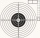The shooter shoots at the target, assuming that the individual shots are independent of each other and the probability of hitting each of them is 0.2. The shooter fires until he hits the target for the first time, then stop firing. (a) What is the most li
• Goat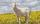Meadow is a circle with radius r = 19 m. How long must a rope to tie a goat to the pin on the perimeter of the meadow to allow goat eat half of meadow?
• V-belt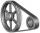Calculate the length of the belt on pulleys with diameters of 105 mm and 393 mm at shaft distance 697 mm.
• The towerThe observer sees the base of the tower 96 meters high at a depth of 30 degrees and 10 minutes and the top of the tower at a depth of 20 degrees and 50 minutes. How high is the observer above the horizontal plane on which the tower stands?
• Cone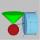Into rotating cone with dimensions r = 8 cm and h = 8 cm incribe cylinder with maximum volume so that the cylinder axis is perpendicular to the axis of the cone. Determine the dimensions of the cylinder.
• Paper boxHard rectangular paper has dimensions of 60 cm and 28 cm. The corners are cut off equal squares and the residue was bent to form an open box. How long must be side of the squares to be the largest volume of the box?
• Traffic laws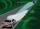Under traffic regulations, car lights can illuminate the road up to a maximum of 30 m. To check the reach of the dipped-beam lights of their car, Peter stopped car at 1.5 m from the wall. The dipped-beam headlights are 60 cm high. At what height on the wa